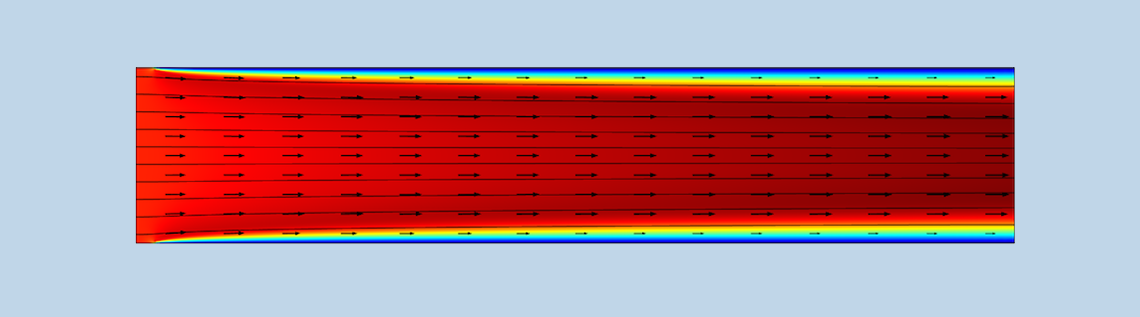# How to Assign Fluid Pressure in CFD Simulations

December 30, 2016

There are many ways to experimentally measure pressure in a fluid. When setting up a CFD model, it is important to use the correct definition of pressure for assigning boundary conditions and defining the material properties. Today, we’ll explain the difference between relative and absolute pressure, why the COMSOL Multiphysics® software solves CFD problems using relative pressure, and when to use each definition of pressure in your models.

### What Is the Difference Between Absolute and Relative Pressure?

In fluid mechanics, pressure represents the force per unit area applied to a surface by a fluid. Using COMSOL Multiphysics, we can solve the governing equations for fluid flow, the Navier-Stokes equations, to determine the velocity and pressure fields that describe the flow.

There are two main ways that we can talk about pressure for CFD problems: absolute pressure and relative pressure.

#### Absolute Pressure

Absolute pressure is the direct measurement of a fluid’s pressure against vacuum. For instance, if we measure the pressure outside on a typical day with a barometer, we see an absolute pressure reading of about 1 atm or 101.325 kPa, which is the atmospheric pressure at sea level. An absolute pressure of zero corresponds to vacuum.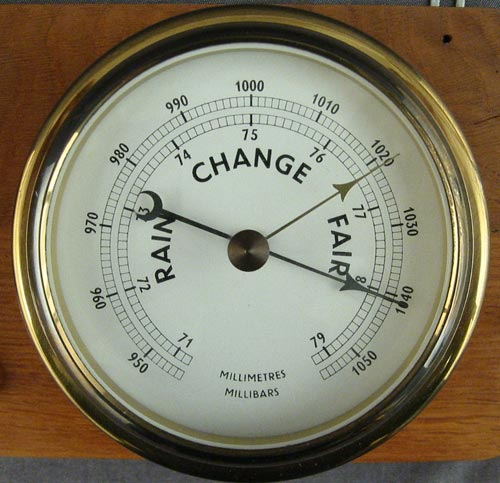This barometer measures the outdoor pressure from 950 to 1050 mbar (1 mbar = 100 Pa). Image by Langspeed. Licensed under CC BY-SA 3.0, via Wikimedia Commons.

#### Relative Pressure

Relative pressure refers to a fluid’s pressure with respect to a reference pressure level. Gauge pressure is the pressure measured relative to ambient pressure; i.e., the relative pressure using ambient pressure as a reference. Typically, relative pressure is used to characterize the pressure levels in closed systems. It can be measured using a manometer, which relates the internal pressure to the surrounding pressure.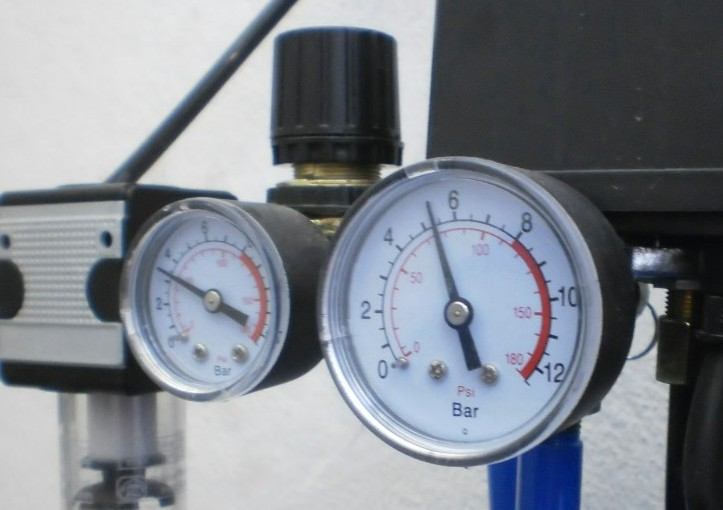Manometers measuring the relative pressure in a pressure control station. Notice how the dials start at zero, which represents the system pressure equaling the reference pressure level. Image by Holmium — Own work. Licensed under CC BY-SA 3.0, via Wikimedia Commons.

Absolute pressure and relative pressure are related according to:

pA = p + pref

If we use vacuum as the reference pressure, then the absolute pressure and the relative pressure are equal. In most cases, the reference pressure is set to the atmospheric pressure, which usually is the ambient pressure.

Let’s connect these definitions of pressure to what we see in COMSOL Multiphysics. When we compute the solution to a fluid flow problem, the COMSOL® software solves for the components of velocity (u,v,w) and the relative pressure (p). As we explain later in this blog post, by using the relative pressure instead of the absolute pressure as the dependent variable, we can improve the accuracy of the description of pressure in our simulation. We can then use the values of relative pressure in the initial values and boundary conditions in the model, which we will see in the following example.

### Characterizing Fluid Pressure in COMSOL Multiphysics®

Let’s take a look at an example that illustrates how to use the variables for relative pressure and absolute pressure appropriately in a COMSOL Multiphysics model. To demonstrate these concepts, we use a simple model of air flowing into a channel with an inlet velocity of 1 m/s and exiting to an absolute outlet pressure of 1 atmosphere. The top and bottom boundaries represent the no-slip channel walls, except for two short inlet sections where we assume symmetry. The inlet sections are there to avoid inconsistent boundary conditions, which would be the case if we were to define a straight inlet velocity profile adjacent to a no-slip boundary.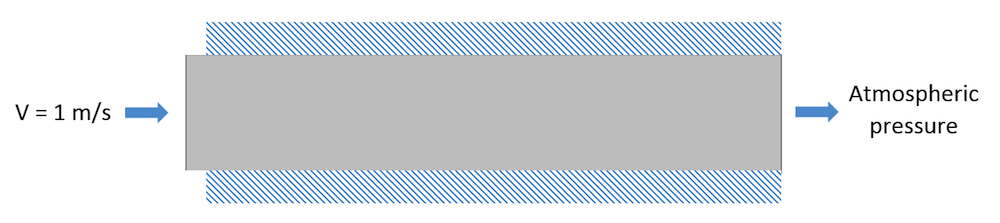A schematic of a channel with air flowing through it.

In this model, the variable name for relative pressure is p and the variable name for absolute pressure is spf.pA. In the settings for the Laminar Flow interface, we see that the dependent variables to be solved are the components of velocity (u,v,w) and the relative pressure (p).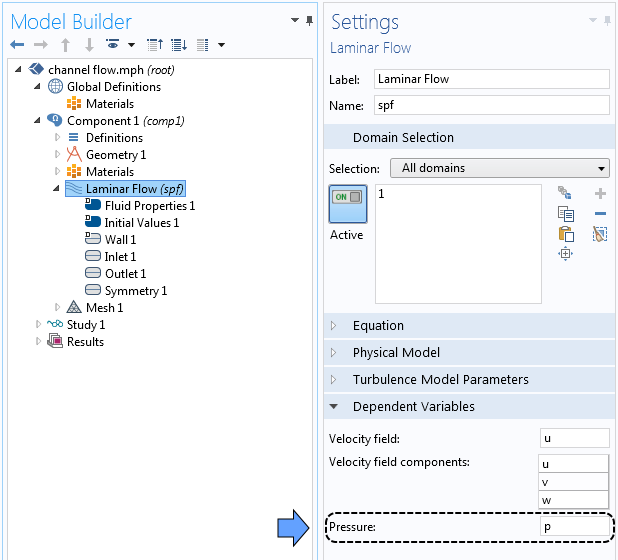Settings window for the dependent variables.

We can see in the figure below that the reference pressure level is set to 1[atm] by default. This reference pressure level is used to calculate the absolute pressure: spf.pA = p + spf.pref.

We also set the compressibility to Weakly compressible flow, which means that the density of air depends on temperature and reference pressure. To learn more about different compressibility settings, take a look at this previous blog post.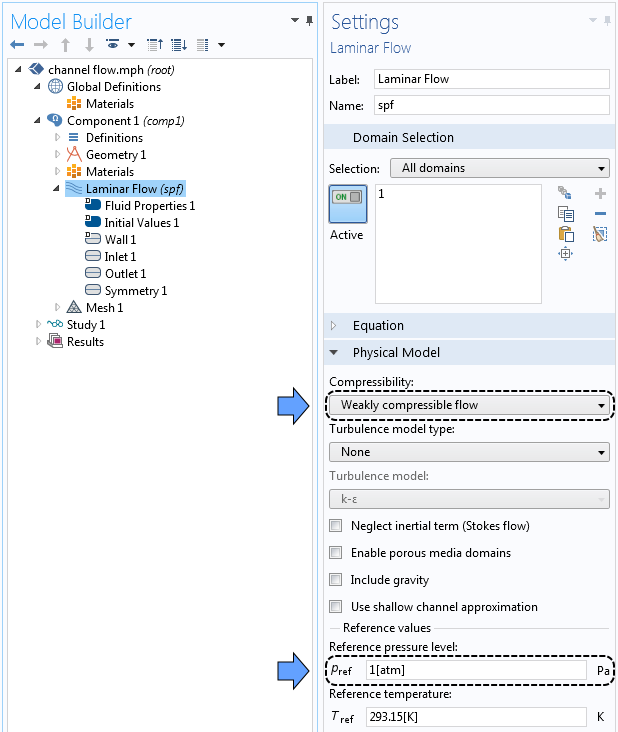The compressibility and reference pressure settings.

Now we can specify our boundary conditions. At the inlet, we assign the normal velocity to be 1 m/s. For the initial conditions and outlet boundary condition, we need to enter the relative pressure, since we are using the default setting; i.e., to use a reference pressure. When we add the outlet condition, we see that the default value for the relative pressure is p = 0, which is equal to 1 atm in absolute pressure for the default reference pressure.

Settings window showing the relative pressure for the initial (left) and boundary (right) conditions.

You might be wondering, then, why the COMSOL® software calculates the variable for absolute pressure, spf.pA. The absolute pressure is used when calculating the density of a compressible fluid. For instance, if we navigate to the material properties for the air in our channel, we will see that the density is defined using the ideal gas law, where pA is the absolute pressure and T is the temperature. Since the ideal gas law is calculated based on the absolute pressure, we have to add the reference pressure to the relative pressure (p) to calculate the density. However, in this case, the relative pressure is such a small fraction of the total pressure (0.00025%; see below) that we may as well use the reference pressure to calculate density, which is what we get when using the Weakly compressible flow option. In systems with larger pressure variations, we can select the Compressible flow option.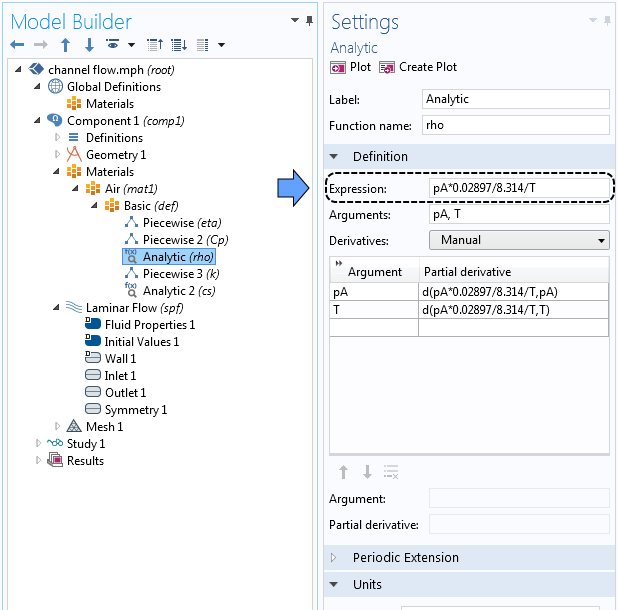Defining density using the ideal gas law in the settings window.

Now that we have defined the boundary conditions for our problem, we can compute the solution and visualize the velocity profile with streamlines.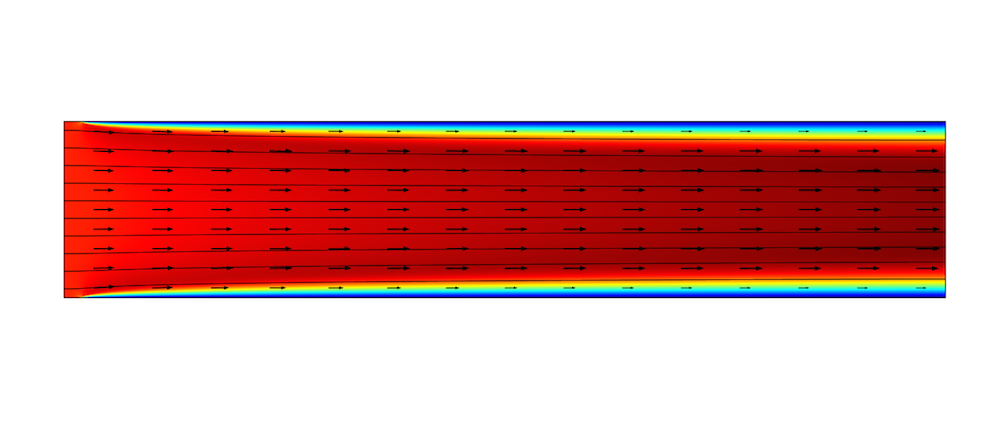Velocity profile with streamlines and a vector plot of flow through a channel.

We can also look at the pressure profile along the inlet (along the y-axis at the left vertical boundary). We can see in the plot below that the pressure variation along the inlet is around one tenth of a Pascal compared to the reference pressure, which is of the order of magnitude of 1·105 Pa. This means that the reference pressure is about one million times larger than the variations in the inlet pressure!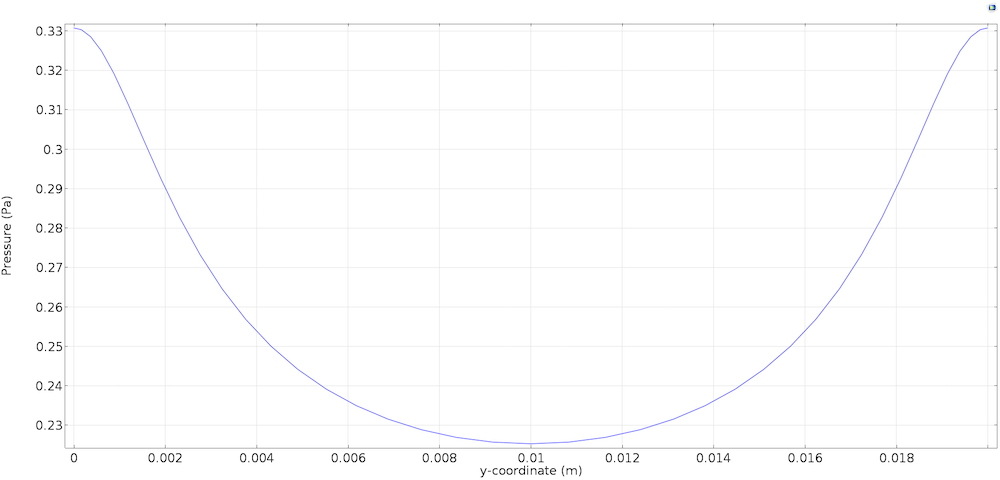Relative pressure along the vertical inlet boundary.

### Solving CFD Problems Using Relative Pressure

The default way to solve fluid flow problems in COMSOL Multiphysics is by using the relative pressure as a dependent variable and adding the reference pressure when an absolute pressure is required; for example, to compute the density of the fluid. This improves the accuracy of the description of the fluctuation of the pressure field around the reference pressure and the description of the gradients of the pressure field.

Let’s return to our channel example and calculate the pressure drop. If we use the Line Average feature to evaluate the relative pressure at the inlet, we will determine the pressure to be about pinlet = 0.26 Pa.

Now, imagine that we solved our problem using absolute pressure instead. The absolute inlet pressure would be 101,325.26 Pa and the absolute outlet pressure would be 101,325.00 Pa. The relative change of the pressure field between the inlet and the outlet is 0.000253814%. As shown in the inlet pressure plot, the variations at the inlet are even smaller: one millionth of the absolute pressure. This is a very small relative change to look for when we solve the equations.

Since we are solving this problem numerically, we are approximating the real pressure field. This is defined at every point, with a numerical approximation defined at a relatively few number of points. We introduce a numerical error due to truncation and interpolation errors. In addition, the numerical equations can only be solved to a given tolerance. This boils down to a relative error in the computed numerical approximation of the pressure field that would disturb the relatively small fluctuations that we are looking for. By using a reference pressure, we can better resolve the gradients of pressure and the fluctuations around atmospheric pressure at viable values for the relative numerical error in the pressure field, compared to the case with the absolute pressure.

### Changing the Reference Pressure Level

Now that we understand why the COMSOL Multiphysics software uses the relative pressure to solve fluid flow problems, we can also appreciate the importance of specifying an accurate reference pressure level. Obviously, a reference pressure level of 1 atm is appropriate for systems working around atmospheric pressure. For very high or low pressure systems, we should use a reference pressure level that matches the pressures expected in the flow.

For instance, in a traditional incandescent light bulb, lower-pressure argon is housed in a glass bulb to prevent oxidation of the filament. In the Application Gallery tutorial model, we see that the reference pressure level changes to match the pressure of this gas (50 kPa). In the Initial Values section, the relative pressure is set to p = 0, which corresponds to an absolute pressure of 50 kPa due to the updated reference pressure level.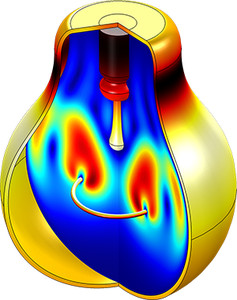A simulation of the free convection of argon within a light bulb.

For very low pressure systems, it’s important to check that fluid can still be considered a continuum. You can calculate the Knudsen number, which is the ratio of the mean free path to the length scale of the device, to determine if the flow is best solved using molecular flow physics.

### Summary on Defining Fluid Pressure in COMSOL Multiphysics®

In today’s blog post, we explained how the absolute pressure of a system is a direct measurement of pressure, while the relative pressure describes the pressure with respect to a reference pressure level.

COMSOL Multiphysics solves CFD problems using relative pressure to improve the numerical accuracy of the pressure field. This means that the initial conditions and boundary condition should be defined using relative pressure values. However, when calculating the density of a gas, the absolute pressure is used and the reference pressure is added to the relative pressure automatically. For high or low pressure systems, the reference pressure level should be changed to match the average pressure in the system.

#### Comments (4)

##### Leave a Comment
Log In | Registration
Loading...##### Aliyuda Ali
November 10, 2017

This is really great, good and perfect. Many thanks to COMSOL Multiphysic team.##### Angela Straccia
November 10, 2017

Aliyuda, thank you for your positive feedback!##### Zhaowang Lin
January 29, 2018

This is a good blog. I have a question about the inlet sections. How do you define the boundary condition of the inlet sections besides “no slip” in order to avoid inconsistent boundary conditions. Thanks!##### Martin Merkel
March 11, 2020

Thank you, that was nicely explained!

I have a question about pressure settings. In my simulation the steam pressure of sodium plays an important role. I thought about to implement the steam pressure as reference pressure but the flow interface does not accept the temperature dependent function.
Is there a possibility to set the reference pressure as a function of temperature? Maybe there is some other way to do this? Thanks.

EXPLORE COMSOL BLOG